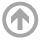Mathematics Department

# Upcoming Talks

This is a list of all upcoming talks for the next two weeks. Talks are from 3:45-4:45 p.m. in the Colloquium or Seminar Room, unless otherwise specified.

• Oct
27
• Statistical reduced-order models and machine learning-based closure strategies for turbulent dynamical systems
Di Qi
Purdue University
Time: 03:45 PM
Colloquium Series

#### View Abstract

The capability of using imperfect statistical reduced-order models to capture crucial statistics in complex turbulent systems is investigated. Much simpler and more tractable block-diagonal models are proposed to approximate the complex and high-dimensional turbulent dynamical equations using both parameterization and machine learning strategies. A systematic framework of correcting model errors with empirical information theory is introduced, and optimal model parameters under this unbiased information measure can be achieved in a training phase before the prediction. It is demonstrated that crucial principal statistical quantities in the most important large scales can be captured efficiently with accuracy using the reduced-order model in various dynamical regimes of the flow field with distinct statistical structures. In addition, new machine learning strategies are proposed to learn the expensive unresolved processes directly from data.
• Nov
03
• RONS: Reduced-order nonlinear solutions for PDEs with conserved quantities
North Carolina State University
Time: 03:45 PM
Colloquium Series

#### View Abstract

Reduced-order models of time-dependent partial differential equations (PDEs) where the solution is assumed as a linear combination of prescribed modes are rooted in a well-developed theory. However, more general models where the reduced solutions depend nonlinearly on time-dependent variables have thus far been derived in an ad hoc manner. I introduce Reduced-order Nonlinear Solutions (RONS): a unified framework for deriving reduced-order models that depend nonlinearly on a set of time-dependent variables. The set of all possible reduced-order solutions are viewed as a manifold immersed in the function space of the PDE. The variables are evolved such that the instantaneous discrepancy between reduced dynamics and the full PDE dynamics is minimized. This results in a set of explicit ordinary differential equations on the tangent bundle of the manifold. In the special case of linear parameter dependence, our reduced equations coincide with the standard Galerkin projection. Furthermore, any number of conserved quantities of the PDE can readily be enforced in our framework. Since RONS does not assume an underlying variational formulation for the PDE, it is applicable to a broad class of problems. I demonstrate its applications on a few examples including the nonlinear Schrodinger equation and Euler's equation for ideal fluids.
• Nov
05
• TBA
Kostya Medynets
USNA Math
Time: 12:00 PM
Applied Math Seminar